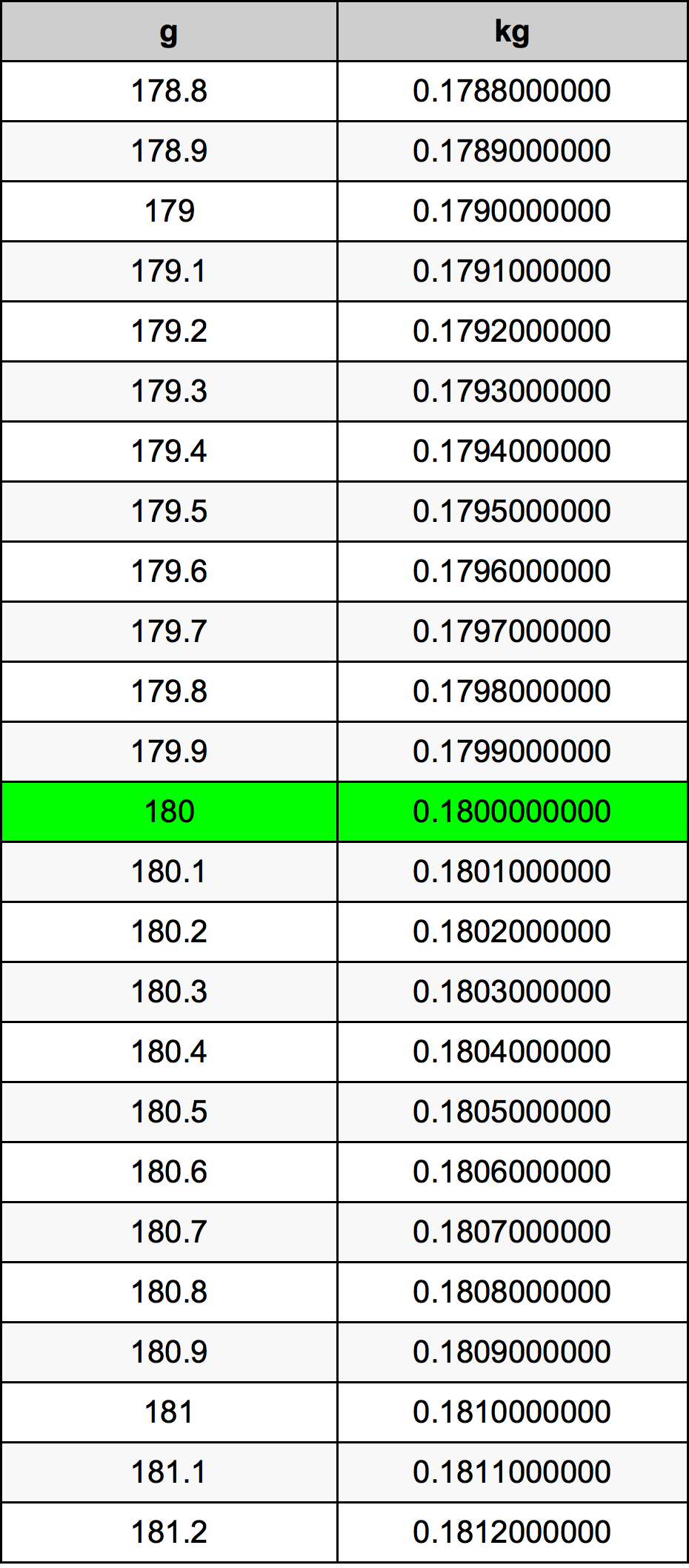Grams To Kilograms

# 180 g to kg180 Grams to Kilograms

g
=
kg

## How to convert 180 grams to kilograms?

 180 g * 0.001 kg = 0.18 kg 1 g
A common question is How many gram in 180 kilogram? And the answer is 180000.0 g in 180 kg. Likewise the question how many kilogram in 180 gram has the answer of 0.18 kg in 180 g.

## How much are 180 grams in kilograms?

180 grams equal 0.18 kilograms (180g = 0.18kg). Converting 180 g to kg is easy. Simply use our calculator above, or apply the formula to change the length 180 g to kg.

## Convert 180 g to common mass

UnitMass
Microgram180000000.0 µg
Milligram180000.0 mg
Gram180.0 g
Ounce6.3493131509 oz
Pound0.3968320719 lbs
Kilogram0.18 kg
Stone0.028345148 st
US ton0.000198416 ton
Tonne0.00018 t
Imperial ton0.0001771572 Long tons

## What is 180 grams in kg?

To convert 180 g to kg multiply the mass in grams by 0.001. The 180 g in kg formula is [kg] = 180 * 0.001. Thus, for 180 grams in kilogram we get 0.18 kg.

## 180 Gram Conversion Table## Alternative spelling

180 Grams to Kilograms, 180 Grams in Kilograms, 180 Gram to Kilogram, 180 Gram in Kilogram, 180 Grams to Kilogram, 180 Grams in Kilogram, 180 Gram to kg, 180 Gram in kg, 180 Gram to Kilograms, 180 Gram in Kilograms, 180 g to kg, 180 g in kg, 180 g to Kilogram, 180 g in Kilogram